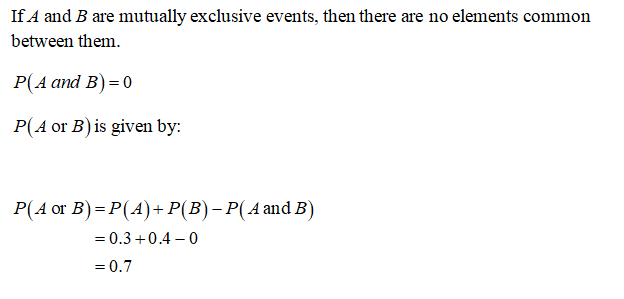# Given P(A) = 0.3 and P(B) = 0.4, do the following.(a) If A and B are mutually exclusive events, compute P(A or B). (b) If P(A and B) = 0.2, compute P(A or B).

Question
8 views

Given P(A) = 0.3 and P(B) = 0.4, do the following.

(a) If A and B are mutually exclusive events, compute
P(A or B).

(b) If P(A and B) = 0.2, compute P(A or B).

check_circle

Step 1...

### Want to see the full answer?

See Solution

#### Want to see this answer and more?

Solutions are written by subject experts who are available 24/7. Questions are typically answered within 1 hour.*

See Solution
*Response times may vary by subject and question.
Tagged in

### Statistics